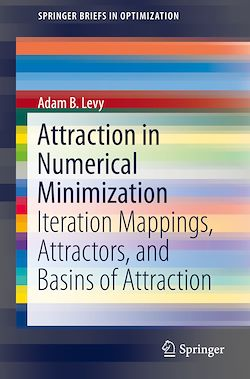78 Pages
English

# Attraction in Numerical Minimization

-Description

Numerical minimization of an objective function is analyzed in this book to understand solution algorithms for optimization problems. Multiset-mappings are introduced to engineer numerical minimization as a repeated application of an iteration mapping. Ideas from numerical variational analysis are extended to define and explore notions of continuity and differentiability of multiset-mappings, and prove a fixed-point theorem for iteration mappings. Concepts from dynamical systems are utilized to develop notions of basin size and basin entropy.  Simulations to estimate basins of attraction, to measure and classify basin size, and to compute basin are included to shed new light on convergence behavior in numerical minimization.

Graduate students, researchers, and practitioners in optimization and mathematics who work theoretically to develop solution algorithms will find this book a useful resource.

Subjects

##### Classwork

Informations